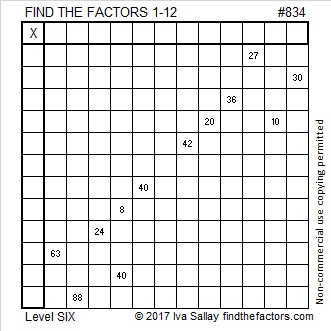# 834 and Level 6834 is the sum of consecutive prime numbers two different ways:

• 127 + 131 + 137 + 139 + 149 + 151 = 834; that’s six consecutive primes
• 31 + 37 + 41 + 43 + 47 + 53 + 59 + 61 + 67 + 71 + 73 + 79 + 83 + 89 = 834; that’s fourteen consecutive primes

The ONLY Pythagorean triple that contains the number 834 is 834 – 173888 – 173890.

• 834 is a composite number.
• Prime factorization: 834 = 2 × 3 × 139
• The exponents in the prime factorization are 1, 1, and 1. Adding one to each and multiplying we get (1 + 1)(1 + 1)(1 + 1) = 2 × 2 × 2 = 8. Therefore 834 has exactly 8 factors.
• Factors of 834: 1, 2, 3, 6, 139, 278, 417, 834
• Factor pairs: 834 = 1 × 834, 2 × 417, 3 × 278, or 6 × 139
• 834 has no square factors that allow its square root to be simplified. √834 ≈ 28.879058There was a solar eclipse in the United States today. People where I lived were able to experience 91.32% obstruction of the sun. I love this interactive map of today’s eclipse and past and future ones as well.

Several people have taken and shared marvelous pictures of the eclipse.